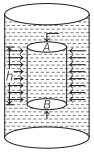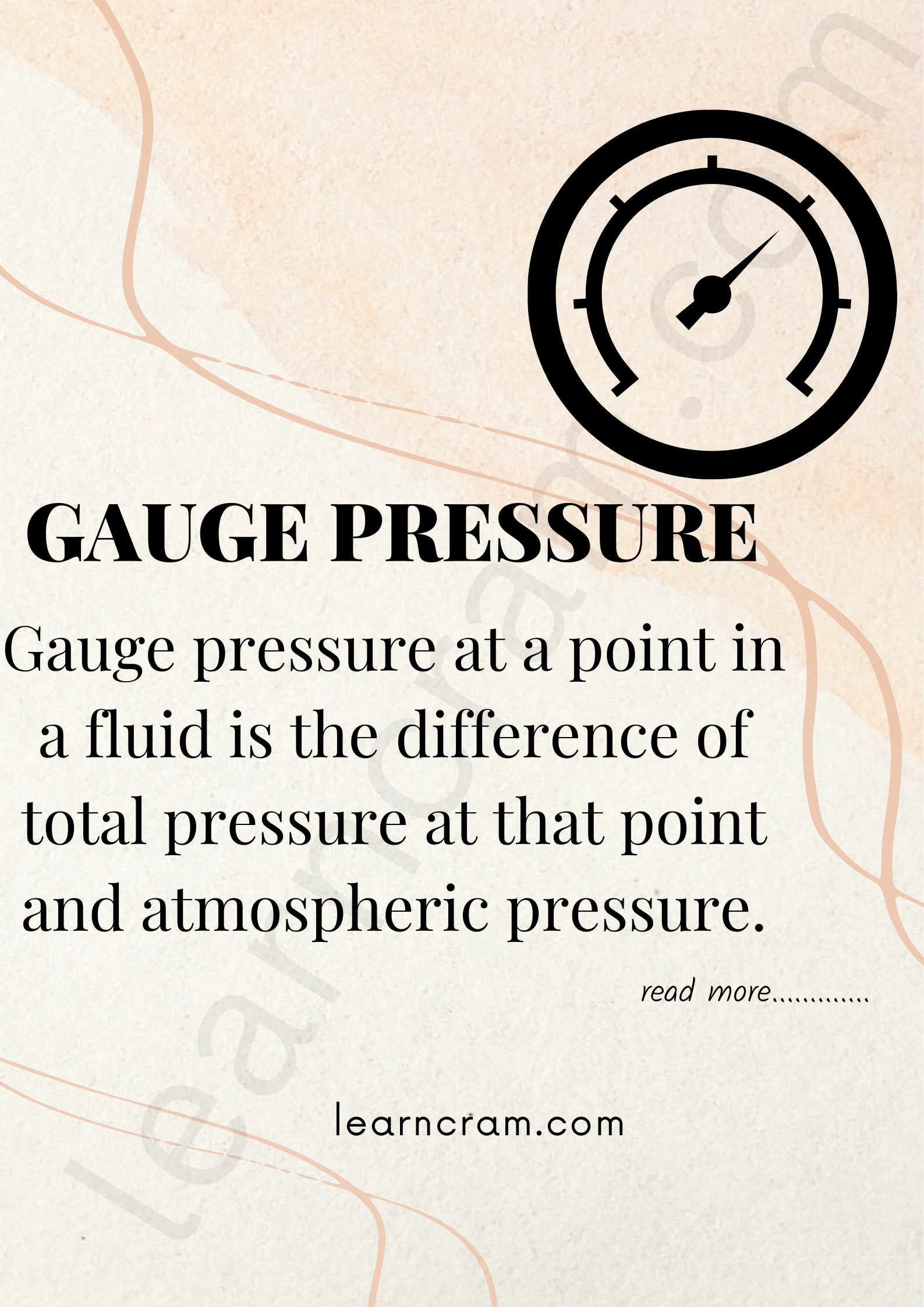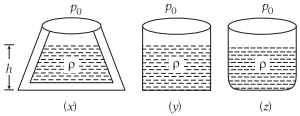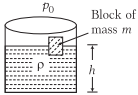# Pressure Exerted by the Liquid – Hydrostatics

Pressure Exerted by the Liquid:
The normal force exerted by a liquid per unit area of the surface in contact is called pressure of liquid or hydrostatic pressure.

We are giving a detailed and clear sheet on all Physics Notes that are very useful to understand the Basic Physics Concepts.

## Pressure Exerted by the Liquid – Hydrostatics

Pressure exerted by a liquid column, p = hρg
where,
h = height of liquid column,
ρ = density of liquid
g = acceleration due to gravity.
Mean pressure on the walls of a vessel containing liquid upto height h is $$\left(\frac{h ρ g}{2}\right)$$.

Variation of Pressure with Depth:
Consider a fluid at rest having density ρ (roh) contained in a cylindrical vessel as shown in figure. Let the two points A and B separated by a vertical distance h.The pressure p at depth below the surface of a liquid open is given by
Pressure, p = pa + hpg
where,
ρ = density of liquid and
g = acceleration due to gravity.

Atmospheric Pressure:
The pressure exerted by the atmosphere on earth is called atmospheric pressure.

• It is equivalent to a weight of 10 tones on 1 m².
• At sea level, atmospheric pressure is equal to 76 cm of mercury column.

Then, atmospheric pressure

= hdg = 76 x 13.6 x 980 dyne/cm²
= 0.76 x 13.6 x 103 x 9.8 N/m²

Thus, 1 atm = 1.013 x 105 Nm-2 (or Pa)

The atmospheric pressure does not crush our body because the pressure of the blood flowing through our circulatory system is balanced by this pressure.

Atmospheric pressure is also measured in torr and bar.

1 torr = 1 mm of mercury column
1 bar = 105 Pa

• Aneroid barometer is used to measure atmospheric pressure.
• Pressure measuring devices are open tube manometer, tyre pressure gauge, sphygmomanometer etc.

Gauge Pressure:
Gauge pressure at a point in a fluid is the difference of total pressure at that point and atmospheric pressure.The liquid pressure at a point is independent of the quantity of liquid but depends upon the depth of point below the liquid surface. This is known as hydrostatic paradox.

### Important Points Related with Fluid Pressure

Important points related with fluid pressure are given below
(i) At a point in the liquid column, the pressure applied on it is same in all directions.

(ii) In a liquid, pressure will be same at all points at the same level.

(iii) The pressure exerted by a liquid depends only on the height of fluid column and is independent of the shape of the containing vessel.If hA = hB = hC, then pA = pB = pC

(iv) Consider following shapes of vesselsPressure at the base of each vessel
Px = Py = Pz = P0 + ρgh but wx ≠ wy ≠ wz
where,
ρ = density of liquid in each vessel,
h = height of liquid in each vessel and
p0 = atmospheric pressure.

(v) In the figure, a block of mass ‘m’ floats over a fluid surfaceIf ρ = density of the liquid
A = area of the block
Pressure at the base of the vessel in p = p0 + ρgh + $$\frac{m g}{A}$$

Hydrostatics:
Hydrostatics is a property of liquid or fluid in mechanics. A fluid is a material which flows at room temperature, because its upper molecule overlaps the inner molecule, which tends to flow the liquid in forward direction. In hydrostatics, we will learn about the condition of fluids when it is in rest or exerted by an external force. Here we will study the fluids in motion.